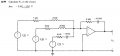#### hsazerty2

Joined Sep 25, 2015
28
Hello,
I am reading a book on circuit analysis: Schaum's Outline of Basic Circuit Analysis, on page 274, Exercice 12.99, we have this circuit :The chapter is about Basic AC circuit analysis;
I know how to get the answer, which is sinusoidal without DC component (as shown above), but when i simulate this circuit (with LTSpice and Proteus 8) i am getting a sinusoid, that's OK, but it has a DC component, around -4v ? is it normal ? if yes, how can we find this DC component analyticaly ?

Thanks.

#### WBahn

Joined Mar 31, 2012
26,398

#### hsazerty2

Joined Sep 25, 2015
28
I first tried 2 DC voltage sources, to get +15v and -15v; then i used an ideal AmpOP component without power rails shown on the schematic, you have to enter inside the properties and change the voltage values: i got the same results; i tried other values, like +12v and -12v; same thing.

I also changed the duration of the analysis, like up to 10 seconds, i always get a nice sinusoid but slightly shifted down (about -4v).

My question is: if l really build this circuit, and i measure Vo, do i get a sinusoid with a DC component ?

#### Jony130

Joined Feb 17, 2009
5,251
but when i simulate this circuit (with LTSpice and Proteus 8) i am getting a sinusoid, that's OK, but it has a DC component, around -4v ? is it normal ? if yes, how can we find this DC component analyticaly ?
Yes, that's normal becomes you do not bias your op amp properly. For DC your opamp work with open loop. Try to add a large resistor between Vout and inverting input.

•hsazerty2

#### WBahn

Joined Mar 31, 2012
26,398
I first tried 2 DC voltage sources, to get +15v and -15v; then i used an ideal AmpOP component without power rails shown on the schematic, you have to enter inside the properties and change the voltage values: i got the same results; i tried other values, like +12v and -12v; same thing.

I also changed the duration of the analysis, like up to 10 seconds, i always get a nice sinusoid but slightly shifted down (about -4v).

My question is: if l really build this circuit, and i measure Vo, do i get a sinusoid with a DC component ?
Probably -- your circuit has no DC feedback (I missed that when I took a quick look before).

In mathematical terms, you opamp is acting as an integrator and when you perform integration you pick up an arbitrary constant, right? So any DC offset you observe is simply one acceptable solution to the differential equation that describes the system. You need to break that ambiguity by putting in some DC feedback between the output and the input. A high-value resistor in parallel with the existing feedback chain should do it.

•hsazerty2

#### hsazerty2

Joined Sep 25, 2015
28it works ! i added a 10 MOhms resistor in parallel with the existing feedback.

So, if i understand :
In DC, the OpAmp is in open loop, so even the slightest voltage at its inputs will produce a measurable DC output. So finally, Vo is the sum of the sinusoidal output (the one shown) and the DC output.

Corrrect me if i am wrong.

Thank you all for your help